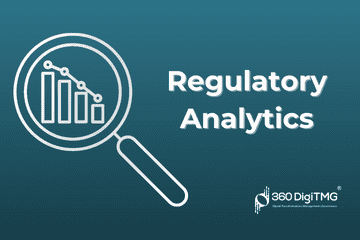Home / Blog / Artificial Intelligence / Naive Bayes

# Naive Bayes

• July 14, 2022
• 5458
• 47### Meet the Author : Mr. Bharani Kumar

Bharani Kumar Depuru is a well known IT personality from Hyderabad. He is the Founder and Director of Innodatatics Pvt Ltd and 360DigiTMG. Bharani Kumar is an IIT and ISB alumni with more than 17 years of experience, he held prominent positions in the IT elites like HSBC, ITC Infotech, Infosys, and Deloitte. He is a prevalent IT consultant specializing in Industrial Revolution 4.0 implementation, Data Analytics practice setup, Artificial Intelligence, Big Data Analytics, Industrial IoT, Business Intelligence and Business Management. Bharani Kumar is also the chief trainer at 360DigiTMG with more than Ten years of experience and has been making the IT transition journey easy for his students. 360DigiTMG is at the forefront of delivering quality education, thereby bridging the gap between academia and industry.

## Introduction

A supervised machine learning approach called Naive Bayes Classifier is used to forecast a non-numeric class. It is commonly recognised as a probabilistic classifier since the Bayes theorem is used to predict the class using the conditional probability idea. Compared to other models, it is a powerful classification model that produces predictions in less time. On the assumption that the characteristics are independent of one another, the Naive Bayes algorithm operates.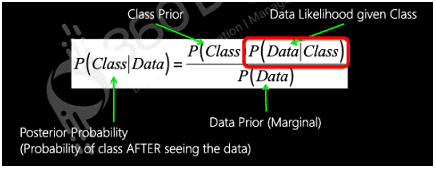Class Prior: P(Class) = Probability of the class in the complete dataset.

Data Likelihood given Class: P(Data|Class) = Probability of the required data in the class

Data Prior: Probability of the data in the complete dataset.

### Gaussian Naïve Bayes:

When dealing with continuous quantities whose probabilities may be represented by a Gaussian distribution, this approach is suitable.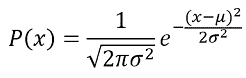### Multinomial Naïve Bayes:

This model is applied for feature vectors where each vector has n different elements which can take k different possible values then we can calculate the probability using multinomial distribution.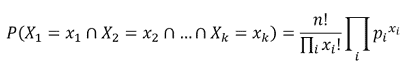### Bernoulli Naïve Bayes:

A random variable's distribution may be determined using the Bernoulli distribution if there are only two potential values for it.### Analysis of the Misclassified Records:

Once, the model has been built, we can analyse the accuracy and error using a contingency matrix which speaks about the correct and incorrect predictions.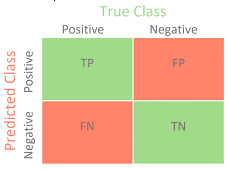Image Source: towardsdatascience

TP: True Positives: This metric indicates the number of instances where positive data was correctly predicted as positive.

FP: False Positives: This metric indicates the number of instances where negative data was incorrectly predicted as positive.

FN: False Negatives: This metric indicates the number of instances where positive data was incorrectly predicted as negative.

TN: True Negatives: This metric indicates the number of instances where negative data was correctly predicted as negative.

Accuracy: This metric is calculated by measuring the proportion of the correctly predicted values in the total number of predictions.
Accuracy=(TP+TN)/(TP+TN+FP+FN)

Error: This metric is calculated by measuring the proportion of the incorrectly predicted values in the total number of predictions.
Accuracy=(FP+FN)/(TP+TN+FP+FN)

Precision: This metric is used for identifying the proportion of True Positives in the total number of positive predictions.
Precision=TP/(TP+FP)

Sensitivity (Recall or Hit Rate or True Positive Rate): This metric is used for identifying the proportion of True Positives in the total number of actually positive instances.
Sensitivity=TP/(TP+FN)

Specificity (True Negative Rate): This metric is used for identifying the proportion of True Negatives in the total number of negative predictions.
Specificity=TN/(TN+FP)

Alpha or Type I error (False Positive Rate): This metric is applied for identifying the incorrectly predicted False Positive values in the total number of negative predictions.
a=1-Specificity

Beta or Type II error (False Negative Rate): This metric is applied for identifying the incorrectly predicted False Negative values in the total number of actually positive instances.
b=1-Sensitivity

F1 Score: The F1 rate indicates the harmonic mean and balance between precision and recall. It can assume values between 0 to 1 which indicate the level of balance maintained between precision and recall.
F1 Score=2 x ((Precision x Recall)/(Precision + Recall))

### Zero Probability Problem:

The main drawback of the Naive Bayes algorithm is that if a class label and an attribute value are unavailable, the probability that the given class of data exists will be zero, and if this specific probability value is multiplied by other probabilities, the result will also be zero. Therefore, we smooth categorical data using Laplace to solve this issue. Conditional probability may be expressed as follows after Laplace smoothing: where k is the number of distinct values of y, and A is the number of distinct values of a. The smoothing constant in this case is l=a, which by default takes on the value 1. If an is zero, then no smoothing has been done.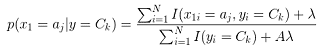Where k represents the number of unique values of y and A represents the number of unique values in a. Here, l=a is the smoothing constant which by default assumes the value 1. If a=0, then it indicates that no smoothing is applied.

### Implementation of Naïve Bayes using Python:

Step 1: Import the necessary modules and the dataset.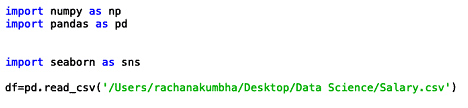### Preview of Dataset: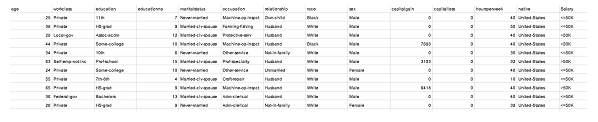Step 2: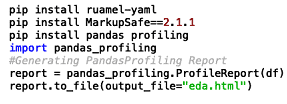### Profile Report: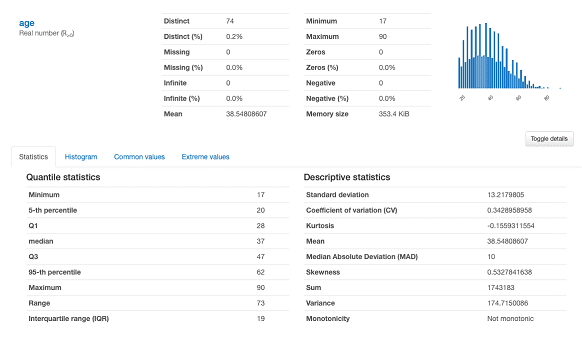Summary of age: We can understand that the minimum age of the working population in this dataset is 17 years and the maximum age is 90 years. Most of the people are of lesser age group and hence it is right-skewed. The mean ages is 38.5 years. There are 74 unique age groups and age has no missing values. Click here to learn Data Science Course in BangaloreSummary of Work class: We can see that there are 7 categories of work classes and most of the people (73.7%) work in the private sector. Also, less than 0.1% of the people work without pay.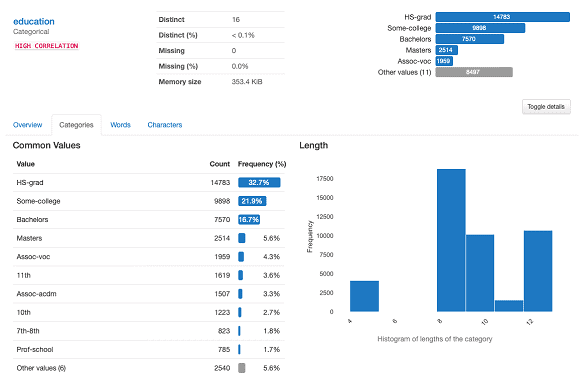Summary of Education: We can see that the highest number of people are only High School graduates (32.7%). However, there are significant number of people who have done Bachelors or attended some college.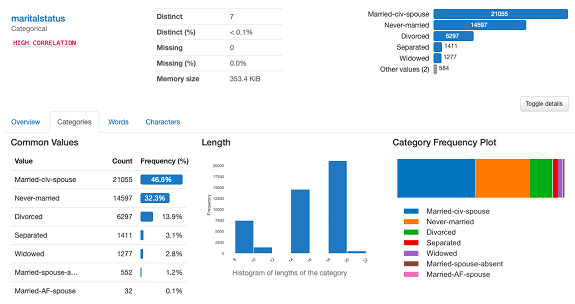Summary of marital status: We can see that there are 7 distinct categories of marital status and most of the people are married (46.6%) or never married (32.3%). There are a very few divorced, separated or other category people in our dataset.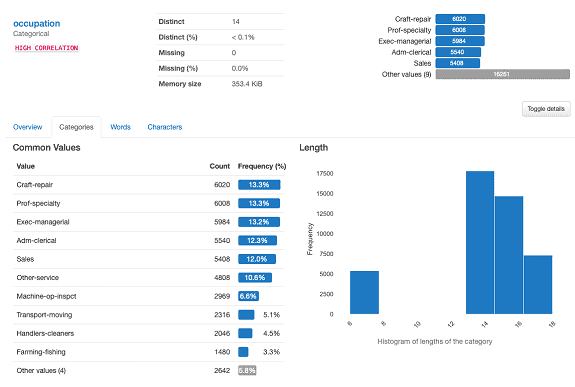Summary of Occupation: We can see that the dataset contains almost equal people in craft-repair and proficient in some specialty. We also a good amount of people belonging to the executive managerial sector, clerical administration and sales. Click here to learn Data Science Training in Hyderabad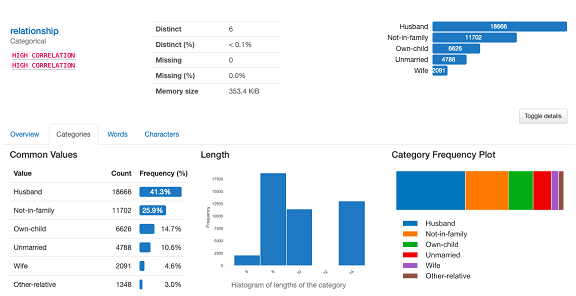Summary of Relationship: We can see that 41.3% of the people have mentioned their husband and also a significant group of people mentioned not in family (25.9%) in our dataset.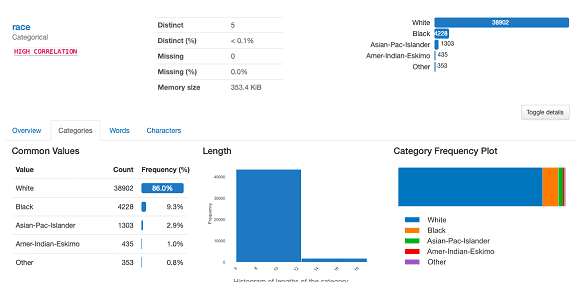Summary of Race: We can see that our dataset contains 86% White population and less percentage of people from other races. Hence, this data is majorly about White people.Summary of Capital Gain: We can observe that the minimum capital gain is 0 and maximum is 99999. However, we can see that 91.6% of the people mentioned 0 as the capital gain. This is a classic example of the Excessive Zeros Model data.Summary of Gender: We can see that there are 67.5% males in the workforce and only 32.5% females. Hence our dataset majorly consists of male population.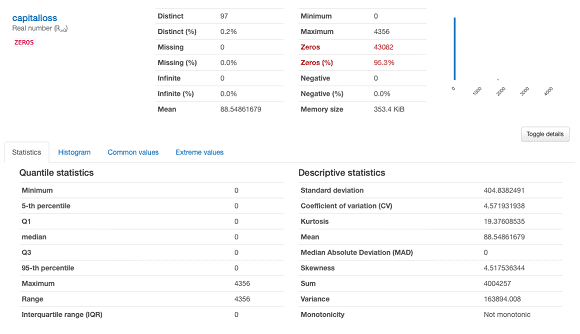Summary of Capital Loss: We can observe that despite the minimum capital loss being 0 and maximum being 4356, the mean capital loss is very less 88.54 which is due to excessive zeros as 95.3% of the people mentioned capital loss as 0. Click here to learn Data Science Course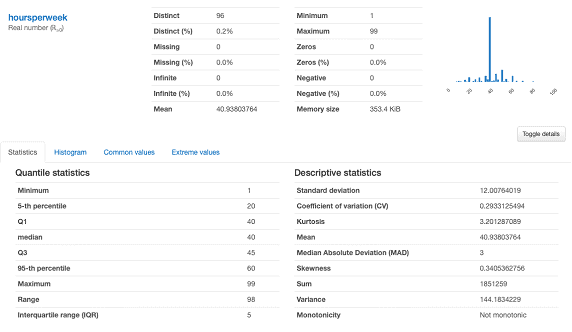Summary of hours per week: We can observe that on an average people are working for 40 hours per week with minimum being 1 hour per week (maybe outlier) and maximum being 99 hours per week. It looks like a symmetric curve(mean=median=mode=40) with most of the people working 40 hours per week.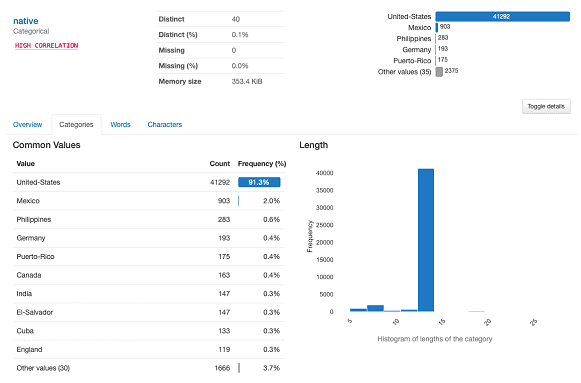Summary of Native: This dataset majorly consists of people of United States nationality where 91.3% of the people are native Americans.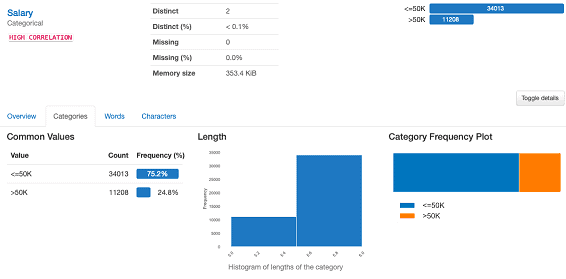Summary of Salary: We can observe that 75.2% of the people are getting salary less than 50,000\$ per annum and only 24.8% people are getting more than 50,000\$ per annum.

Step 3: Data Cleaning: After analyzing our data by performing Exploratory Data Analysis(EDA), we need to clean our data before building our final model.

3.1 Missing value Handling: After verifying for missing data, we found none in any of the columns, which is also consistent with the profile report.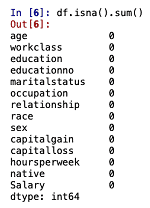3.2 Duplicates Handling: While checking for duplicates using the relevant function, we can observe that there are 5982 duplicates and after dropping the duplicates, we can recheck that there are 0 duplicate values. Click here to learn Data Science Course in Chennai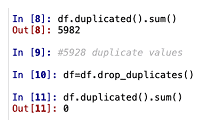3.3 Outlier Treatment: Using a boxplot, extreme values and outliers may be seen. Utilising the Winsorizer function, they may be handled. We may perform a second check using the boxplot after handling the outliers with the Winsorizer function. (See the code and boxplots below.)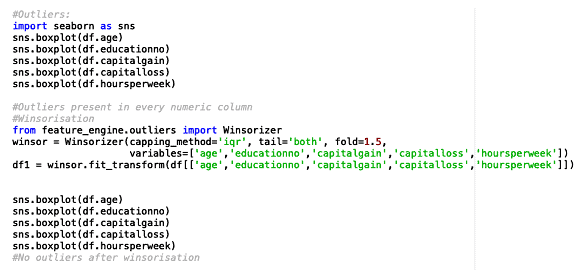### Boxplot before and after Outlier Treatment: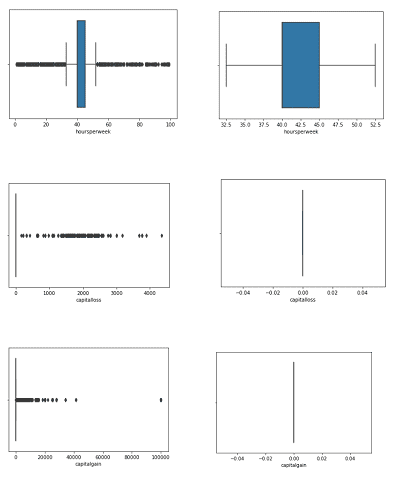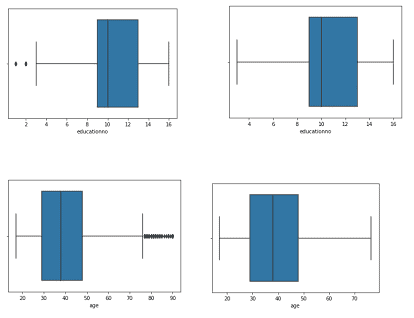3.4 Dummy Variable Creation: Since, our dataset consists of non-numeric data, we can convert them to numeric data by creating dummy variables. We can use OneHotEncoder function to convert nominal to binary data and LabelEncoder function to convert ordinal to interval data.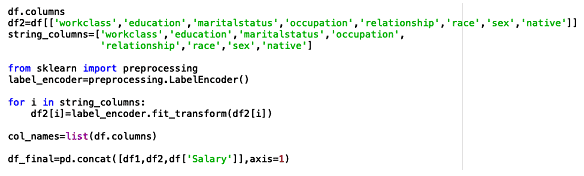### Preview of Dataset after Cleaning: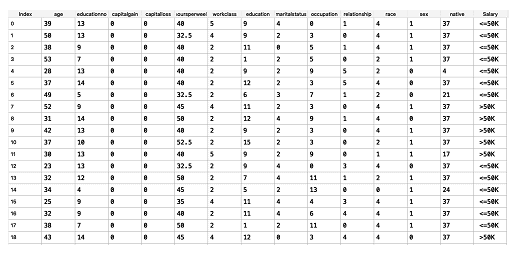Step 4: After all of the data has been thoroughly cleaned, we can go on to the Model Building step, but first we must separate the data into predictors and targets in order to develop our model. Since our ultimate goal is to predict pay, we will use the "Salary" column as our objective and all the variables affecting income as our predictors. To create predictions once the training is over, we must further divide the data into Training data and Test data. We are utilising 80% of the data for training the model and 20% for testing in the code below.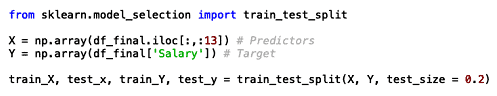### Step 5: Model Building and Evaluation

5.1 Gaussian Naïve Bayes Model: If any of our inputs are continuous and follow the Gaussian Distribution, we may apply this model. By comparing the real and projected numbers, we are also assessing the code's train and test correctness. If the actual value matches the projected value, we will consider it True (1); otherwise, we will consider it False (0). By calculating the mean of the True values, the accuracy is determined. To create our Gaussian Naive Bayes Model, we'll be utilising the GaussianNB function from the sklearn.naive_bayes module.Output: We can observe that the training accuracy is 77.64% and the test accuracy is 77.37%. We can see a huge number of False Negatives (1135) and False Positives (641) by comparing the actual and predicted values using the confusion matrix.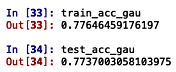Classification Analysis: We can observe that the number of True Positives is 4682 and True Negatives is 1390 which is contributing to the accuracy of the model. However, the number of False Negatives is 1135 and False Positives are 641 which means that 1135 positive values are predicted as negative and 641 negative values are predicted as positive. Due to the high False Negative Rate or b error, we can say that the model is not very good. Also, we can observe that the aggregated f1-score is 0.77 which indicates only 77% test accuracy.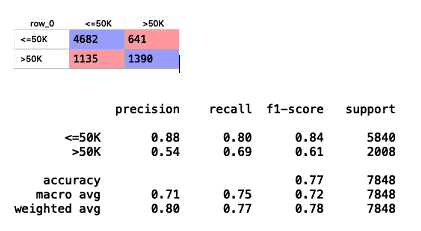5.2 Multinomial Naïve Bayes Model: If the target data is text or non-numeric, we utilise this approach a lot. Using the MultinomialNB function from the sklearn.naive Bayes package, we can create a Multinomial Naive Bayes Model.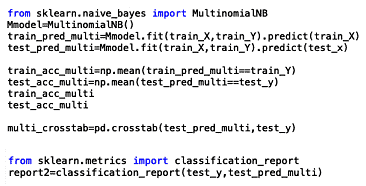Output: We can observe that both the training accuracy (72.88%) and the test accuracy (72.12%) of the Multinomial Naïve Bayes model are lesser than the Gaussian Naïve Bayes model though the number of False Negatives (1300) are lesser for Multinomial Naïve Bayes model.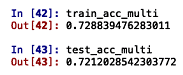Classification Analysis: We can observe that the number of True Positives are 4517 and True Negatives are 1149 which is contributing to the accuracy of the model. However, the number of False Negatives are 1300 and False Positives are 888 which means that 1300 positive values are predicted as negative and 888 negative values are predicted as positive. Hence, we can say that the  error is higher than Gaussian model. Also, we can observe that the aggregated f1-score is 0.72 which indicates only 72% test accuracy.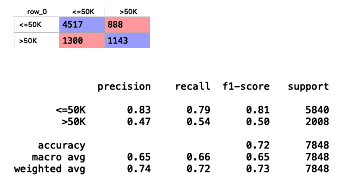5.3 Bernoulli’s Naïve Bayes Model: Usually, if the predictors are binary in nature, this model is employed. The BernoulliNB function from the sklearn.naive_bayes module may be used to create this model.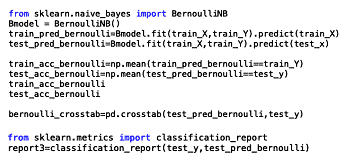Output: We can observe that the training accuracy (73.22%) and the test accuracy(73.21%) for Bernouilli’s model are higher than the Multinomial Naïve Bayes Model but lesser than the Gaussian Naïve Bayes Model. Also, it has the highest number of False Negatives(1516) which suggests that it is not a good model.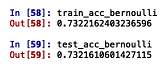Classification Analysis: We can observe that the number of True Positives are 4301 and True Negatives are 1445 which is contributing to the accuracy of the model. However, the number of False Negatives are 1516 and False Positives are 586 which means that 1516 positive values are predicted as negative and 586 negative values are predicted as positive. Hence, we can say that the  error is higher than Gaussian model and Multinomial Naïve Bayes model. Also, we can observe that the aggregated f1-score is 0.73 which indicates only 73% test accuracy which is higher than Multinomial model but lesser than Gaussian Naïve Bayes model.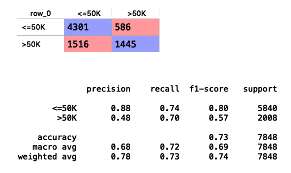5.4 Multinomial Naïve Bayes Model after Laplace Smoothing: Applying Laplace smoothing to categorical data generated by hyperparameter tweaking can help us get over the zero-probability issue mentioned above.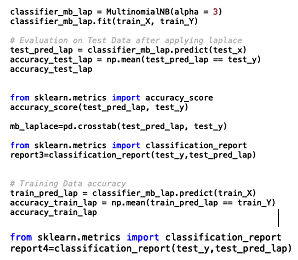Output: We can observe that the training accuracy is 72.66% and test accuracy is 72.64%. Since, the accuracy of the model has decreased, we can try with different values of  and take the best value which gives us a higher accuracy.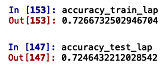Classification Analysis: We can observe that the number of True Positives are 4607 and True Negatives are 1080 which is contributing to the accuracy of the model. However, the number of False Negatives are 1233 and False Positives are 928 which means that 1233 positive values are predicted as negative and 928 negative values are predicted as positive. Hence, we can say that the  error is higher than Gaussian model but lesser than Multinomial or Bernoulli’s model. But the  error is higher than all the models. Also, we can observe that the aggregated f1-score is 0.72 which indicates only 72% test accuracy.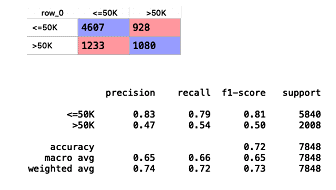Best Model: From our observations, we can conclude that Gaussian Naïve Bayes is a better model in terms of training and test accuracy compared to Bernoulli’s or Multinomial Naïve Bayes models.

• Naïve Bayes is a simple and fast algorithm.
• It needs lesser training data for model building.
• It works well with continuous, discrete, binary or multiclass data.
• It is scalable with increase in the number of predictors.

• The main disadvantage of the Naïve Bayes models is that is assumes that the features are completely independent but in reality it is not possible to have completely independent features in the same dataset.
• Naïve Bayes Classifier also has a ‘Zero Probability’ issue which means that if a categorical variable is not observed in the training dataset though it belongs to a category, then Naïve Bayes will not be able to predict as it will allocate a zero probability to it.

### Applications of Naïve Bayes

• Recommendation Systems
• Spam Filtering
• Sentiment Analysis
• Credit Scoring
• Text Classification

Click here to learn Data Science Course, Data Science Course in Hyderabad, Data Science Course in Bangalore

### Data Science Training Institutes in Other Locations